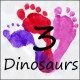Easter Egg Spinning Multiplication & Division Math Facts

I love having no-prep math pages that I can use to review math facts. They make it easy to review them over and over.

Today I have a fun Easter Themed Math fact spinning page for multiplication and division facts!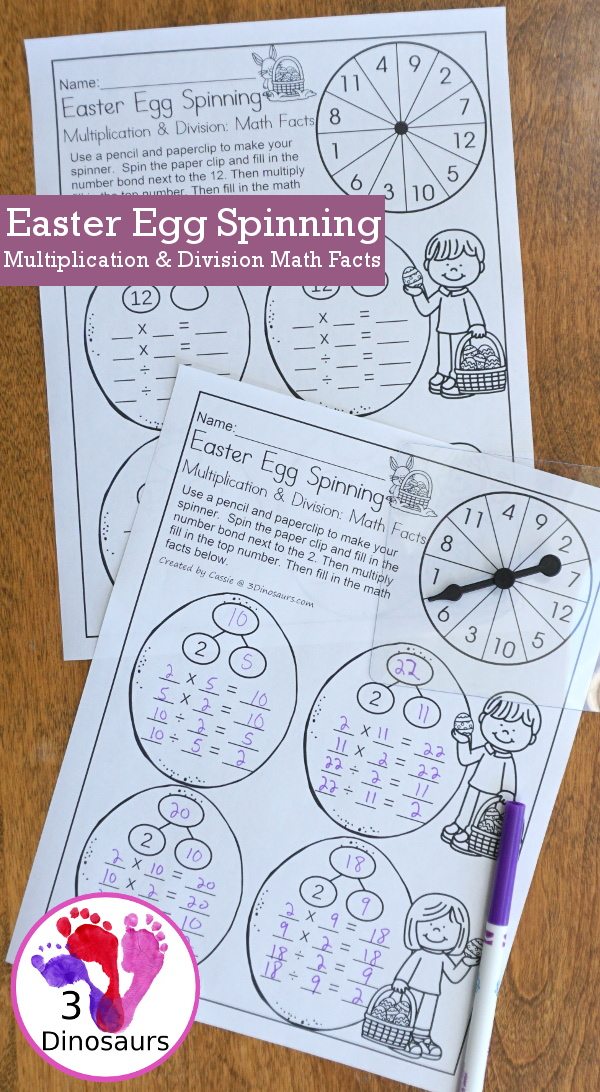I love the different holidays and doing something fun for them. Easter is a great holiday that kids have fun doing activities with eggs. Today’s no-prep math page is great for just that. No-prep and reviewing multiplication and division math facts.

Each of the pages has 4 math facts to fill out per page. The page focuses on one number at a time. It also has a fun spinner so you can use the pages over and over for the math facts. We used the clear spinnerson the spinner. But a pencils and paperclip work as well.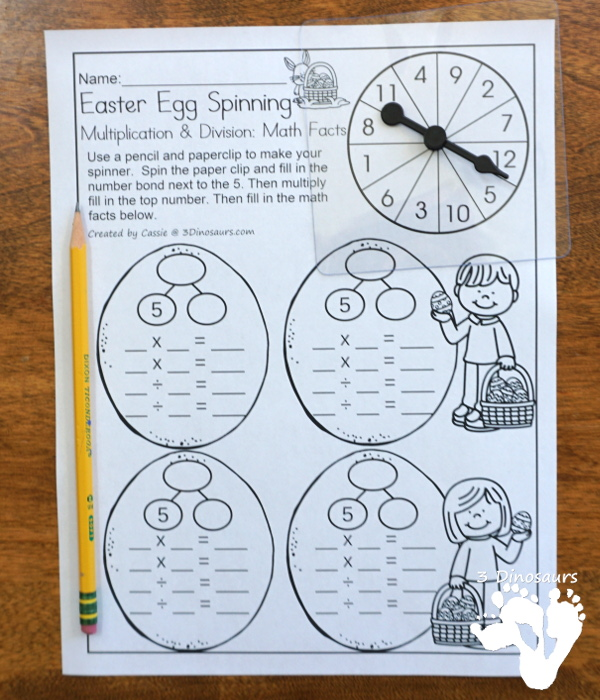How we use this printable

Each of the pages has 1 number to focus on. It has one part of the number bond filled out. With that number. You can see the number 8 filled out on this page. We used the spinner to get the second number to multiply.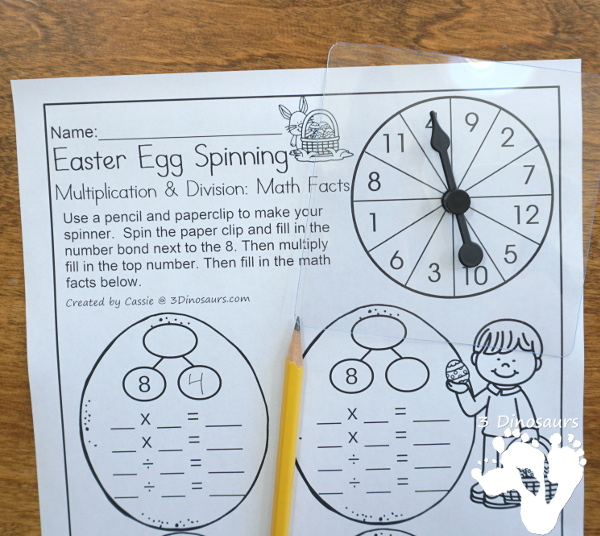Then multiply them together to find the top number and fill out the math facts. If they are having issues with the math fact you can grab the multiplication and division bookmarks to help them.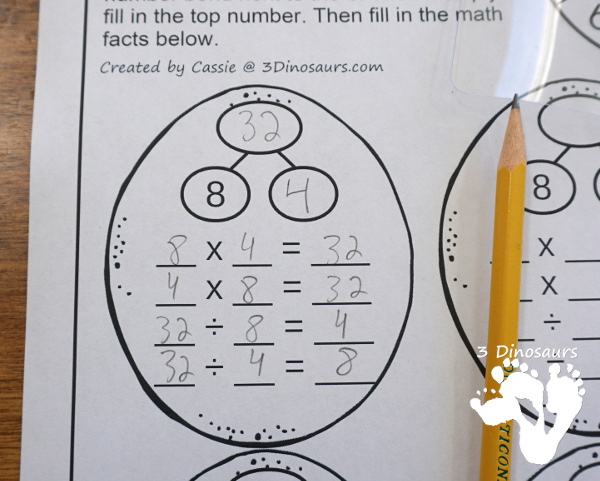Now if you want to use the page over and over then put it in a sheet protector and use dry erase markers on it. Then the sheet can be used as often as you want!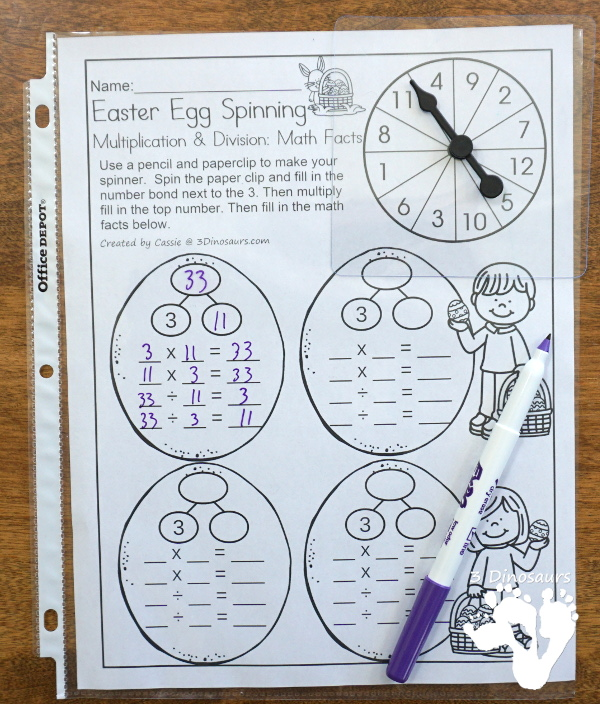If you are looking for some non-seasonal math facts then check out the Multiplication & Division Math Fact House Books! These are great for review or learning math facts.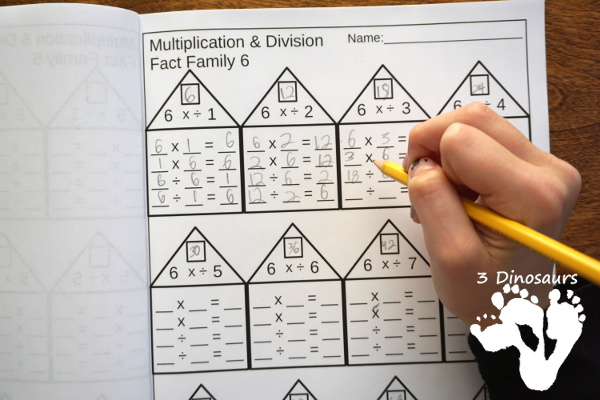What you will find in the Multiplication & Division Math Fact Books printables:

The set is a collection of two types of books: Beginner Multiplication & Division Math Fact Books and Review Multiplication & Division Math Fact Books. Both books give kids a chance to work on learning and reviewing their math fact for multiplication and division.What you will find in these printables:

• Math facts for Multiplication & Division from 1 to 12
• 12 Beginner Multiplication & Division Math Fact Books
• Single Print and Teacher Print Options for the Math Fact Books
• 15 pages for each begginner books
• 3 pages Types for the Review Multiplication & Division Math Fact Books
• Blank
• 1 number of the number bond filled out
• 2 numbers of the number bond filled out
• Option to use as a No-Prep Printable for the Review Multiplication & Division Math Fact Books
• 316 pages of printables
• Price: \$4.50

What we will find in the free Easter Egg Spinning Multiplication & Division Math Facts

• Multiplication and Division for 2 to 12
• Math fact sheet and spinner on each page
• No-prep pages
• 11 pages of printables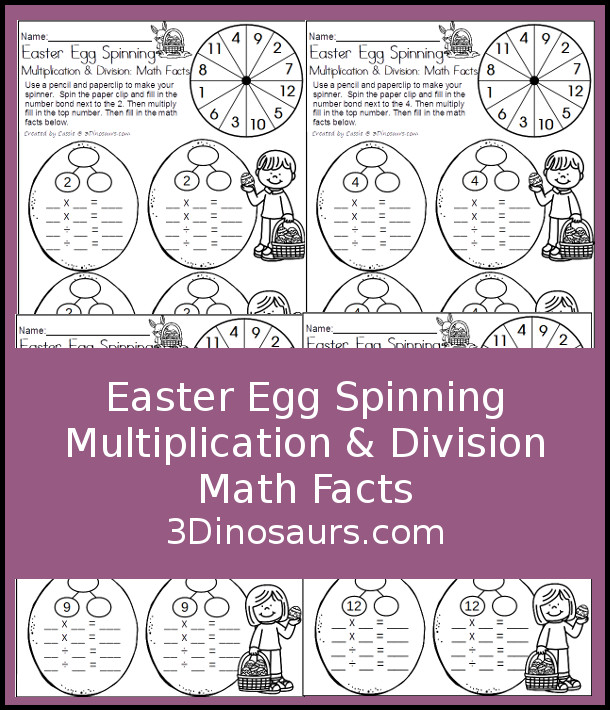Check out all the other Easter Activities and printables on 3 Dinosaurs:

Cassie – 3Dinosaurs.com